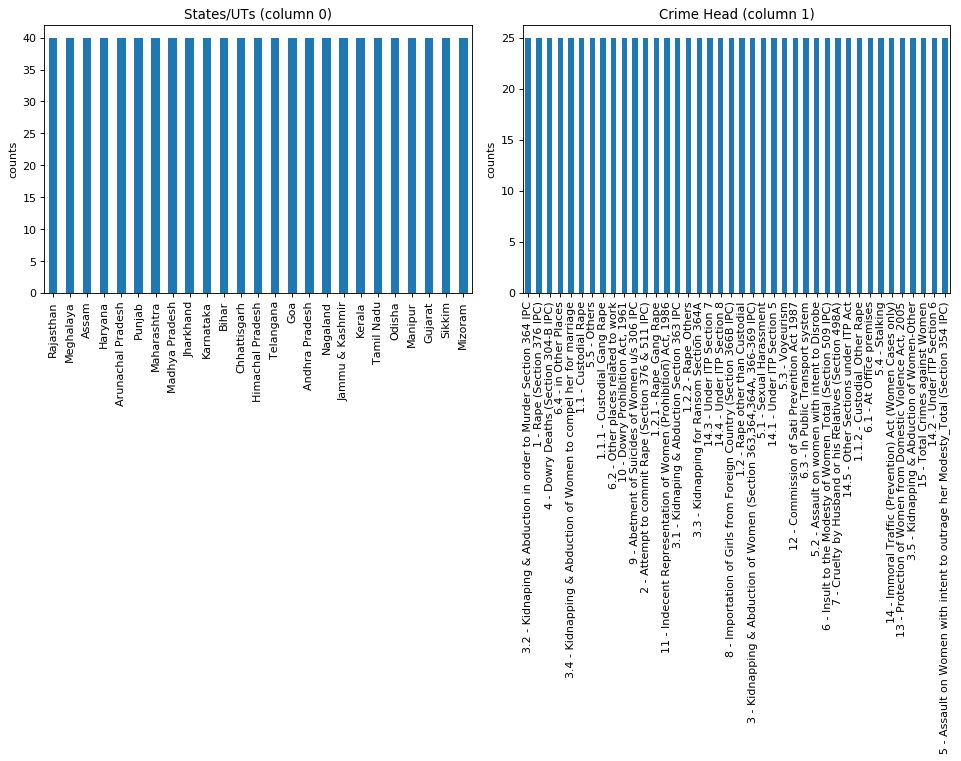### Introduction

Greetings from the Kaggle bot! This is an automatically-generated kernel with starter code demonstrating how to read in the data and begin exploring. Click the blue "Edit Notebook" or "Fork Notebook" button at the top of this kernel to begin editing.

### Exploratory Analysis

To begin this exploratory analysis, first use `matplotlib` to import libraries and define functions for plotting the data. Depending on the data, not all plots will be made. (Hey, I'm just a kerneling bot, not a Kaggle Competitions Grandmaster!)

In :
``````from mpl_toolkits.mplot3d import Axes3D
from sklearn.preprocessing import StandardScaler
import matplotlib.pyplot as plt # plotting
import numpy as np # linear algebra
import os # accessing directory structure
import pandas as pd # data processing, CSV file I/O (e.g. pd.read_csv)

``````

There is 1 csv file in the current version of the dataset:

In :
``````for dirname, _, filenames in os.walk('/kaggle/input'):
for filename in filenames:
print(os.path.join(dirname, filename))

``````
```/kaggle/input/CrimeAgainstWomen.csv ```

The next hidden code cells define functions for plotting data. Click on the "Code" button in the published kernel to reveal the hidden code.

In :
``````# Distribution graphs (histogram/bar graph) of column data
def plotPerColumnDistribution(df, nGraphShown, nGraphPerRow):
nunique = df.nunique()
df = df[[col for col in df if nunique[col] > 1 and nunique[col] < 50]] # For displaying purposes, pick columns that have between 1 and 50 unique values
nRow, nCol = df.shape
columnNames = list(df)
nGraphRow = (nCol + nGraphPerRow - 1) / nGraphPerRow
plt.figure(num = None, figsize = (6 * nGraphPerRow, 8 * nGraphRow), dpi = 80, facecolor = 'w', edgecolor = 'k')
for i in range(min(nCol, nGraphShown)):
plt.subplot(nGraphRow, nGraphPerRow, i + 1)
columnDf = df.iloc[:, i]
if (not np.issubdtype(type(columnDf.iloc), np.number)):
valueCounts = columnDf.value_counts()
valueCounts.plot.bar()
else:
columnDf.hist()
plt.ylabel('counts')
plt.xticks(rotation = 90)
plt.title(f'{columnNames[i]} (column {i})')
plt.tight_layout(pad = 1.0, w_pad = 1.0, h_pad = 1.0)
plt.show()

``````
In :
``````# Correlation matrix
def plotCorrelationMatrix(df, graphWidth):
filename = df.dataframeName
df = df.dropna('columns') # drop columns with NaN
df = df[[col for col in df if df[col].nunique() > 1]] # keep columns where there are more than 1 unique values
if df.shape < 2:
print(f'No correlation plots shown: The number of non-NaN or constant columns ({df.shape}) is less than 2')
return
corr = df.corr()
plt.figure(num=None, figsize=(graphWidth, graphWidth), dpi=80, facecolor='w', edgecolor='k')
corrMat = plt.matshow(corr, fignum = 1)
plt.xticks(range(len(corr.columns)), corr.columns, rotation=90)
plt.yticks(range(len(corr.columns)), corr.columns)
plt.gca().xaxis.tick_bottom()
plt.colorbar(corrMat)
plt.title(f'Correlation Matrix for {filename}', fontsize=15)
plt.show()

``````
In :
``````# Scatter and density plots
def plotScatterMatrix(df, plotSize, textSize):
df = df.select_dtypes(include =[np.number]) # keep only numerical columns
# Remove rows and columns that would lead to df being singular
df = df.dropna('columns')
df = df[[col for col in df if df[col].nunique() > 1]] # keep columns where there are more than 1 unique values
columnNames = list(df)
if len(columnNames) > 10: # reduce the number of columns for matrix inversion of kernel density plots
columnNames = columnNames[:10]
df = df[columnNames]
ax = pd.plotting.scatter_matrix(df, alpha=0.75, figsize=[plotSize, plotSize], diagonal='kde')
corrs = df.corr().values
for i, j in zip(*plt.np.triu_indices_from(ax, k = 1)):
ax[i, j].annotate('Corr. coef = %.3f' % corrs[i, j], (0.8, 0.2), xycoords='axes fraction', ha='center', va='center', size=textSize)
plt.suptitle('Scatter and Density Plot')
plt.show()

``````

Now you're ready to read in the data and use the plotting functions to visualize the data.

#### Let's check 1st file: /kaggle/input/CrimeAgainstWomen.csv

In :
``````nRowsRead = 1000 # specify 'None' if want to read whole file
# CrimeAgainstWomen.csv may have more rows in reality, but we are only loading/previewing the first 1000 rows
df1 = pd.read_csv('/kaggle/input/CrimeAgainstWomen.csv', delimiter=',', nrows = nRowsRead)
df1.dataframeName = 'CrimeAgainstWomen.csv'
nRow, nCol = df1.shape
print(f'There are {nRow} rows and {nCol} columns')``````
```There are 1000 rows and 3 columns ```

Let's take a quick look at what the data looks like:

In :
``df1.head(5)``
Out:

Distribution graphs (histogram/bar graph) of sampled columns:

In :
``plotPerColumnDistribution(df1, 10, 5)``### Conclusion

This concludes your starter analysis! To go forward from here, click the blue "Edit Notebook" button at the top of the kernel. This will create a copy of the code and environment for you to edit. Delete, modify, and add code as you please. Happy Kaggling!